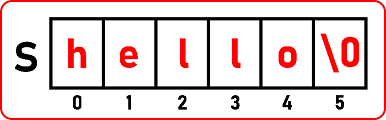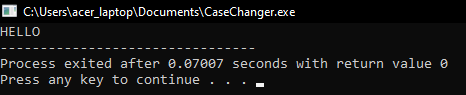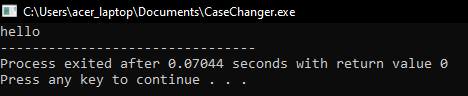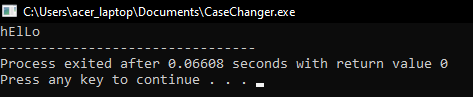# How to Change Case of Alphabets in a String in C

## How to Change Case of Alphabets in a String C Language

In this article, I am going to discuss How to Change the Case of Alphabets in a String C Language with Examples. Please read our previous article where we discussed How to Find the Length of a String in C Language with Examples.

##### How to Change Case of Alphabets in a String in C Language?

In this article, we will see how to change the case of alphabets from lowercase to uppercase or vice versa of characters in a string.Here we have taken a string “hello”. So, all letters are in lowercase. We have to change it to uppercase. We have seen the ASCII code for alphabets:

Uppercase Alphabets: 65 (A) to 90 (Z)

Lowercase Alphabets: 97 (a) to 122 (z)

We know these cases so it means when it is small ‘h’ I have to change it to uppercase ‘H’. So,

1. for small ‘h’ code is 104 and for capital ‘H’ code is 72, the difference is 32.
2. for small ‘e’ code is 101 and for capital ‘E’ code is 69, the difference is 32.
3. for small ‘l’ code is 108 and for capital ‘L’ code is 76, the difference is 32.
4. for small ‘o’ code is 111 and for capital ‘L’ code is 79, the difference is 32.

So, the difference is 32 for every small and capital alphabet. So, it means that uppercase and lowercase letters differences are 32. If any letter is in lowercase and we subtract 32 to that then we will get an uppercase ASCII code.

So, it is possible to add a number to alphabets. Actually, the alphabets or letters are nothing but codes. For a programmer or for the user it is visible as ‘h’ but actually, it is ASCII code inside the main memory. So, we can modify it by adding some numbers.

So how to convert from lowercase to uppercase, subtract 32 let us give it a try. These are the indices starting from 0 onwards, we will subtract 32 from all these alphabets then we will get capital letters.

Let us write the procedure for converting the cases, we have to scan through all these alphabets. For that I need a ‘for’ loop:

##### Lowercase to Uppercase Code in C Language:
#include <stdio.h>
#include <stdlib.h>
int main ()
{
char B[] = “hello”;
int i;
//For lowercase to Uppercase
for (i = 0; B[i] != ‘\0’; i++)
{
B[i] = B[i] – 32;
}
printf (“%s”, B);
}
Output:##### Uppercase to Lowercase Code in C Language:
#include <stdio.h>
#include <stdlib.h>
int main(){
char B[] = “HELLO”;
int i;
//For Uppercase to lowercase
for(i = 0; B[i] != ‘\0’; i++){
B[i] = B[i] + 32;
}
printf(“%s”, B);
}
Output:##### How to Toggle the cases of a string in C language

We have already seen how to scan through a string or how to traverse a string using a ‘for’ loop. Now next thing we will show you is how to toggle the cases. If it is lowercase, then convert it to uppercase and vice versa. Let us look at how to toggle the cases of alphabets in a string.

char S = “HeLlo”;

I have the alphabets above; some are in the uppercase like ‘H’ and ‘L’ and the rest of them are in lower cases. So, whichever are in lowercase we will change them into an uppercase and uppercase to lowercase. So, if it is in uppercase then we have to add 32 and if it is lowercase then we have to subtract 32. For that, we have to scan for this whole string.

##### Toggle the cases of a string Code in C language:
#include <stdio.h>
#include <stdlib.h>
int main(){
char B[] = “HeLlO”;
int i;
for(i = 0; B[i] != ‘\0’; i++){
if(B[i] >= 65 && B[i] <= 90)
B[i] += 32;
else if(B[i] >= ‘a’ && B[i] <= ‘z’){
B[i] -= 32;
}
}
printf(“%s”, B);
}

Output: